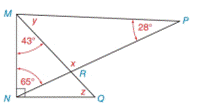Chapter 2.4, Problem 16E### Elementary Geometry for College St...

6th Edition
Daniel C. Alexander + 1 other
ISBN: 9781285195698

#### Solutions

Chapter
Section### Elementary Geometry for College St...

6th Edition
Daniel C. Alexander + 1 other
ISBN: 9781285195698
Textbook Problem
1 views

# Given: M N ¯ ⊥ N Q ¯ and ∠ s as shown Find: x , y , and zTo determine

To find:

The value of x, y, and z.

Explanation

Given:

The given statement is,

MN¯NQ¯.

Figure (1)

Properties:

(1) In a triangle, the sum of the measures of the interior angles is 180°.

(2) If two line segments are perpendicular to each other, they intersect at an angle of 90°

(3) Vertically opposite angles are same in measure.

(4) The measure of an exterior angle of a triangle equals the sum of the measures of the two nonadjacent interior angles

Calculation:

The given statement is,

MN¯NQ¯, MNR=65°, RMN=43° and MPR=28°

So,

MNQ=90°RNQ=MNQMNR

Substitute 90° for MNQ and 65° for MNR in the above equation.

RNQ=90°65°RNQ=25°

In ΔRMN,

mRMN+mMNR+mNRM=180°43°+65°+mNRM=180°mNRM=180°43°65°mNRM=72°

NRM is an exterior angle of ΔRMP.

So,

mNRM=mRMP+mMPR

Substitute y for RMP and 28° for MPR, and 72° for NRM in the above equation

### Still sussing out bartleby?

Check out a sample textbook solution.

See a sample solution

#### The Solution to Your Study Problems

Bartleby provides explanations to thousands of textbook problems written by our experts, many with advanced degrees!

Get Started

#### Find more solutions based on key concepts